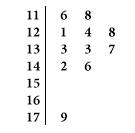### CFA Practice Question

There are 985 practice questions for this topic.

### CFA Practice Question

For a physics course containing 11 students, the maximum point total for the quarter was 200. The point totals for the 11 students are given in the stem plot below.Which of the following statements is (are) true about the stem plot?

I. Because there are 7 stems, the median must be contained in the 4th smallest stem and be between 140 and 150.
II. We can compute the mean number of points for the 11 students from the information in the stem plot.
III. Because of the small sample size, it would be impossible to split the stems in this stem plot.

I. The median must be computed as the middle observation, not the middle stem, and, depending on the shape of the distribution, the middle observation does not necessarily need to be contained in the middle stem.
II. The stem plot gives the individual observations, so it is possible to compute the value of any summary statistics from the stem plot.
III. You can always split the stems, regardless of the sample size. In this example, you wouldn't want to, because there would be too few leaves in each stem, making it difficult to get an overall impression of the shape.